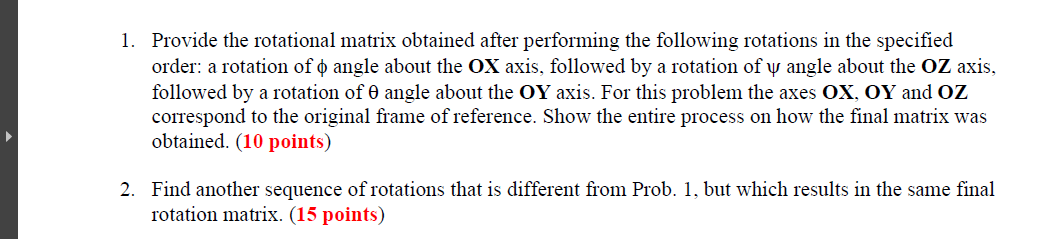Home / Answered Questions / Other / 1-provide-the-rotational-matrix-obtained-after-performing-the-following-rotations-in-the-specified-o-aw301

# (Solved): 1. Provide The Rotational Matrix Obtained After Performing The Following Rotations In The Specified ...1. Provide the rotational matrix obtained after performing the following rotations in the specified order: a rotation of o angle about the OX axis, followed by a rotation of y angle about the OZ axis, followed by a rotation of angle about the OY axis. For this problem the axes OX, OY and OZ correspond to the original frame of reference. Show the entire process on how the final matrix was obtained. (10 points) 2. Find another sequence of rotations that is different from Prob. 1, but which results in the same final rotation matrix. (15 points)

We have an Answer from Expert